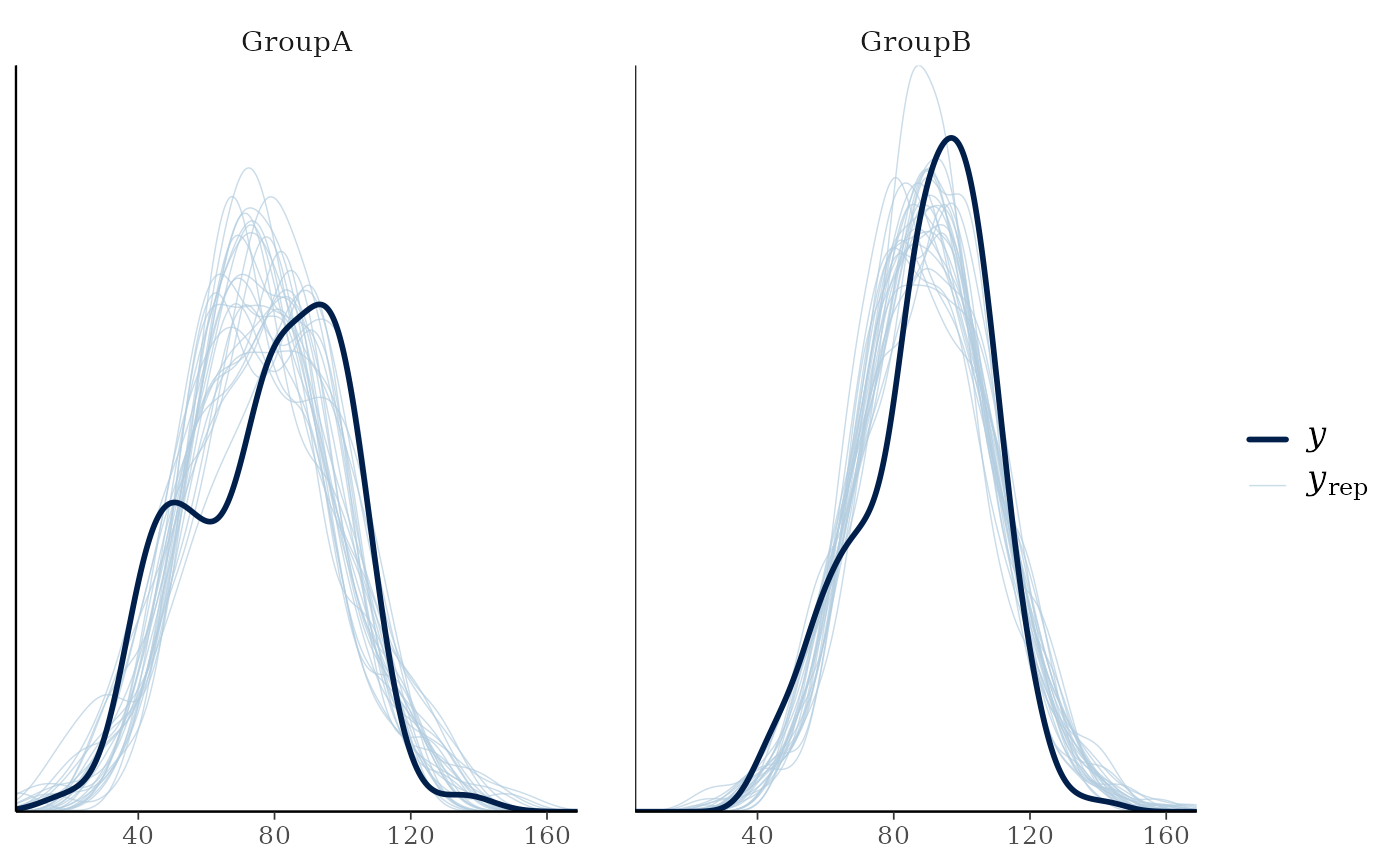Compare the empirical distribution of the data y to the distributions of simulated/replicated data yrep from the posterior predictive distribution. See the Plot Descriptions section, below, for details.

ppc_data(y, yrep, group = NULL)

ppc_hist(y, yrep, ..., binwidth = NULL, breaks = NULL, freq = TRUE)

ppc_boxplot(y, yrep, ..., notch = TRUE, size = 0.5, alpha = 1)

ppc_freqpoly(y, yrep, ..., binwidth = NULL, freq = TRUE, size = 0.25,
alpha = 1)

ppc_freqpoly_grouped(y, yrep, group, ..., binwidth = NULL, freq = TRUE,
size = 0.25, alpha = 1)

ppc_dens(y, yrep, ..., trim = FALSE, size = 0.5, alpha = 1)

ppc_dens_overlay(y, yrep, ..., size = 0.25, alpha = 0.7,
trim = FALSE, bw = "nrd0", adjust = 1, kernel = "gaussian",
n_dens = 1024)

ppc_ecdf_overlay(y, yrep, ..., discrete = FALSE, pad = TRUE,
size = 0.25, alpha = 0.7)

ppc_violin_grouped(y, yrep, group, ..., probs = c(0.1, 0.5, 0.9),
size = 1, alpha = 1, y_draw = c("violin", "points", "both"),
y_size = 1, y_alpha = 1, y_jitter = 0.1)

## Arguments

y A vector of observations. See Details. An $$S$$ by $$N$$ matrix of draws from the posterior predictive distribution, where $$S$$ is the size of the posterior sample (or subset of the posterior sample used to generate yrep) and $$N$$ is the number of observations (the length of y). The columns of yrep should be in the same order as the data points in y for the plots to make sense. See Details for additional instructions. A grouping variable (a vector or factor) the same length as y. Each value in group is interpreted as the group level pertaining to the corresponding value of y. Currently unused. Passed to ggplot2::geom_histogram() to override the default binwidth. Passed to ggplot2::geom_histogram() as an alternative to binwidth. For histograms, freq=TRUE (the default) puts count on the y-axis. Setting freq=FALSE puts density on the y-axis. (For many plots the y-axis text is off by default. To view the count or density labels on the y-axis see the yaxis_text() convenience function.) A logical scalar passed to ggplot2::geom_boxplot(). Unlike for geom_boxplot(), the default is notch=TRUE. Passed to the appropriate geom to control the appearance of the yrep distributions. A logical scalar passed to ggplot2::geom_density(). Optional arguments passed to stats::density() to override default kernel density estimation parameters. n_dens defaults to 1024. For ppc_ecdf_overlay(), should the data be treated as discrete? The default is FALSE, in which case geom="line" is passed to ggplot2::stat_ecdf(). If discrete is set to TRUE then geom="step" is used. A logical scalar passed to ggplot2::stat_ecdf(). A numeric vector passed to ggplot2::geom_violin()'s draw_quantiles argument to specify at which quantiles to draw horizontal lines. Set to NULL to remove the lines. For ppc_violin_grouped(), a string specifying how to draw y: "violin" (default), "points" (jittered points), or "both". For ppc_violin_grouped(), if y_draw is "points" or "both" then y_size, y_alpha, and y_jitter are passed to to the size, alpha, and width arguments of ggplot2::geom_jitter() to control the appearance of y points. The default of y_jitter=NULL will let ggplot2 determine the amount of jitter.

## Value

The plotting functions return a ggplot object that can be further customized using the ggplot2 package. The functions with suffix _data() return the data that would have been drawn by the plotting function.

## Details

For Binomial data, the plots will typically be most useful if y and yrep contain the "success" proportions (not discrete "success" or "failure" counts).

## Plot Descriptions

ppc_hist(), ppc_freqpoly(), ppc_dens(), ppc_boxplot()

A separate histogram, shaded frequency polygon, smoothed kernel density estimate, or box and whiskers plot is displayed for y and each dataset (row) in yrep. For these plots yrep should therefore contain only a small number of rows. See the Examples section.

ppc_freqpoly_grouped()

A separate frequency polygon is plotted for each level of a grouping variable for y and each dataset (row) in yrep. For this plot yrep should therefore contain only a small number of rows. See the Examples section.

ppc_dens_overlay(), ppc_ecdf_overlay()

Kernel density or empirical CDF estimates of each dataset (row) in yrep are overlaid, with the distribution of y itself on top (and in a darker shade). When using ppc_ecdf_overlay() with discrete data, set the discrete argument to TRUE for better results. For an example of ppc_dens_overlay() also see Gabry et al. (2019).

ppc_violin_grouped()

The density estimate of yrep within each level of a grouping variable is plotted as a violin with horizontal lines at notable quantiles. y is overlaid on the plot either as a violin, points, or both, depending on the y_draw argument.

Gabry, J. , Simpson, D. , Vehtari, A. , Betancourt, M. and Gelman, A. (2019), Visualization in Bayesian workflow. J. R. Stat. Soc. A, 182: 389-402. doi:10.1111/rssa.12378. (journal version, arXiv preprint, code on GitHub)

Gelman, A., Carlin, J. B., Stern, H. S., Dunson, D. B., Vehtari, A., and Rubin, D. B. (2013). Bayesian Data Analysis. Chapman & Hall/CRC Press, London, third edition. (Ch. 6)

## See also

Other PPCs: PPC-discrete, PPC-errors, PPC-intervals, PPC-loo, PPC-overview, PPC-scatterplots, PPC-test-statistics

## Examples

color_scheme_set("brightblue")
y <- example_y_data()
yrep <- example_yrep_draws()
dim(yrep)#>  500 434ppc_dens_overlay(y, yrep[1:25, ])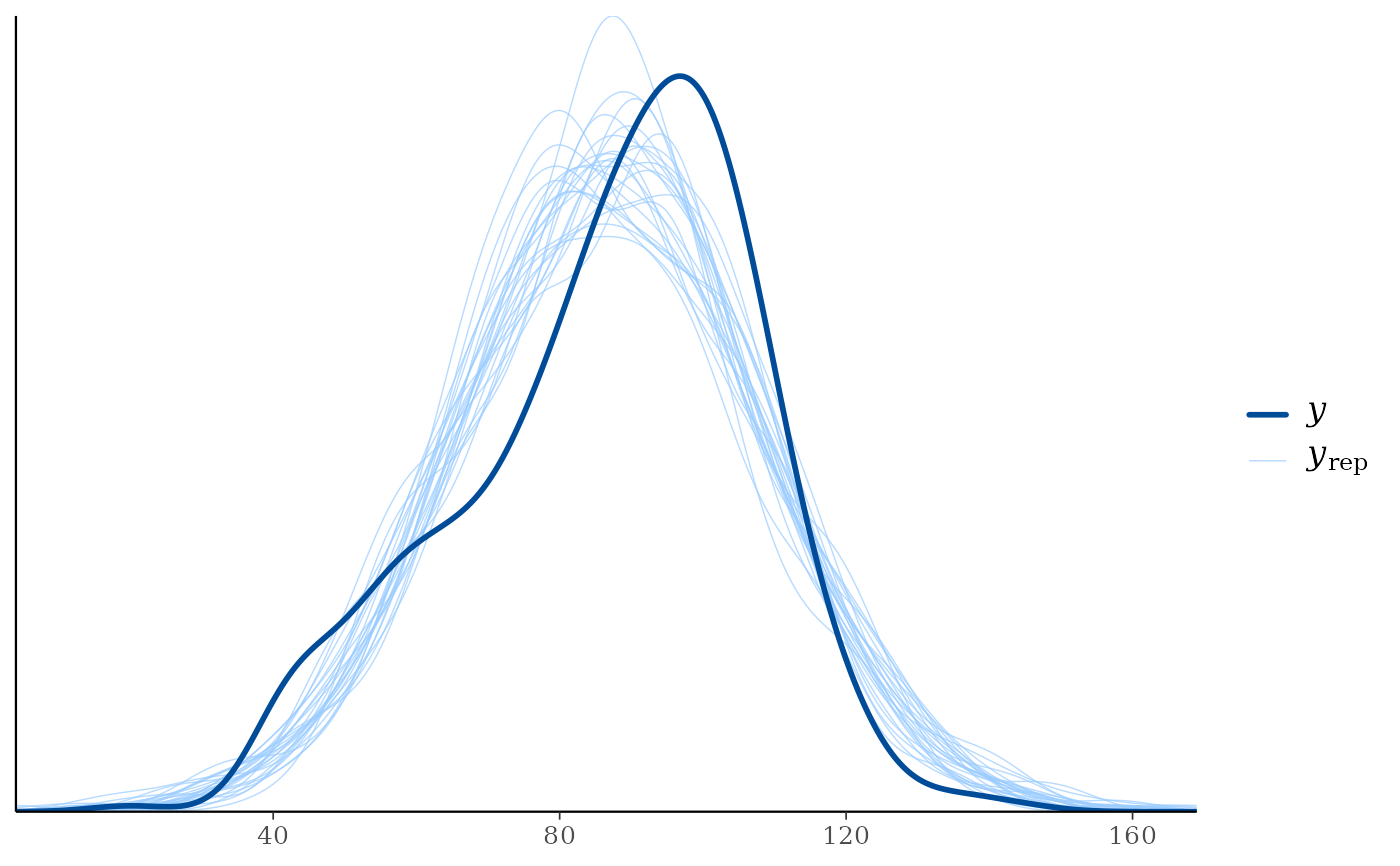ppc_ecdf_overlay(y, yrep[sample(nrow(yrep), 25), ])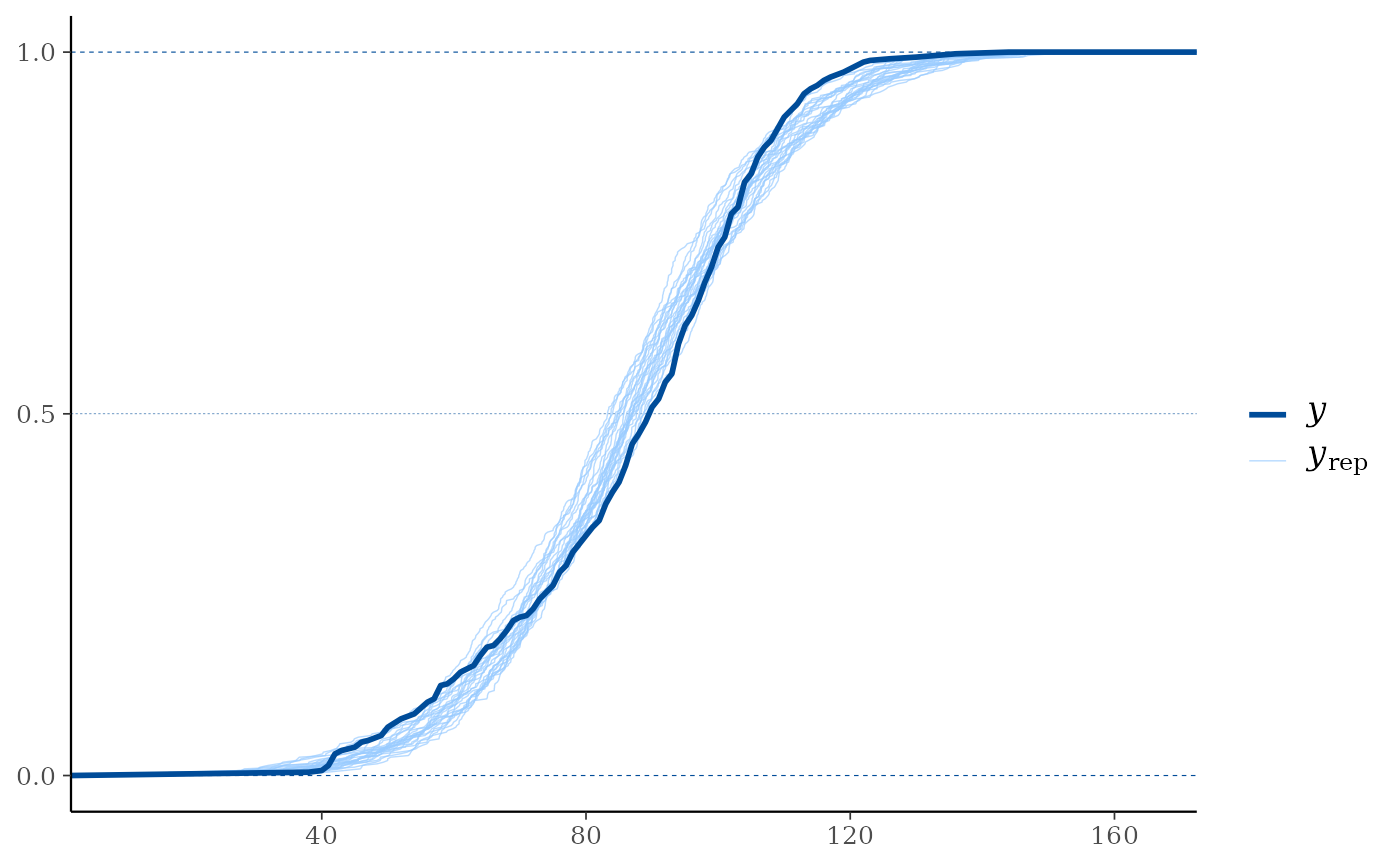# for ppc_hist,dens,freqpoly,boxplot definitely use a subset yrep rows so
# only a few (instead of nrow(yrep)) histograms are plotted
ppc_hist(y, yrep[1:8, ])#> stat_bin() using bins = 30. Pick better value with binwidth.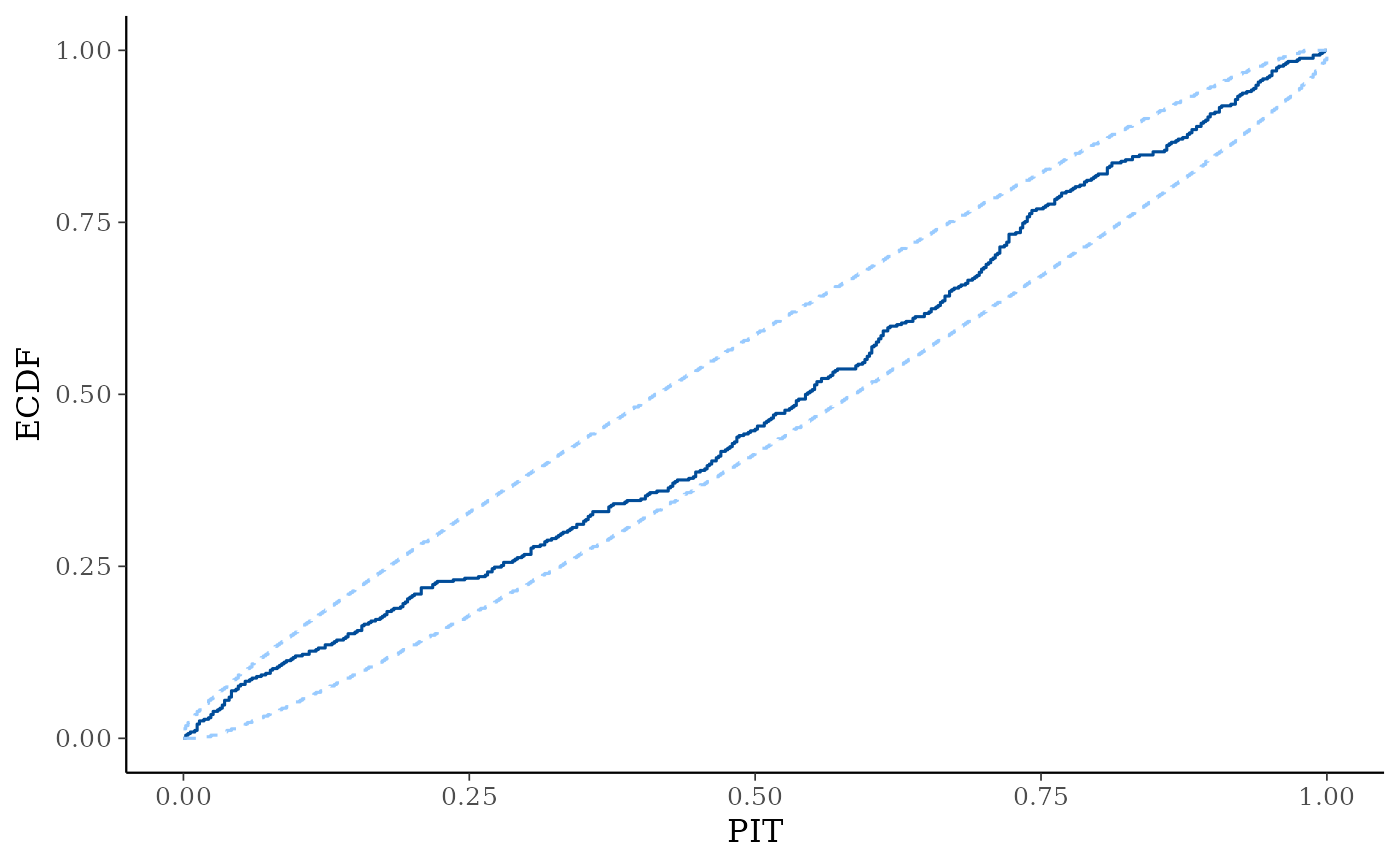color_scheme_set("red")
ppc_boxplot(y, yrep[1:8, ])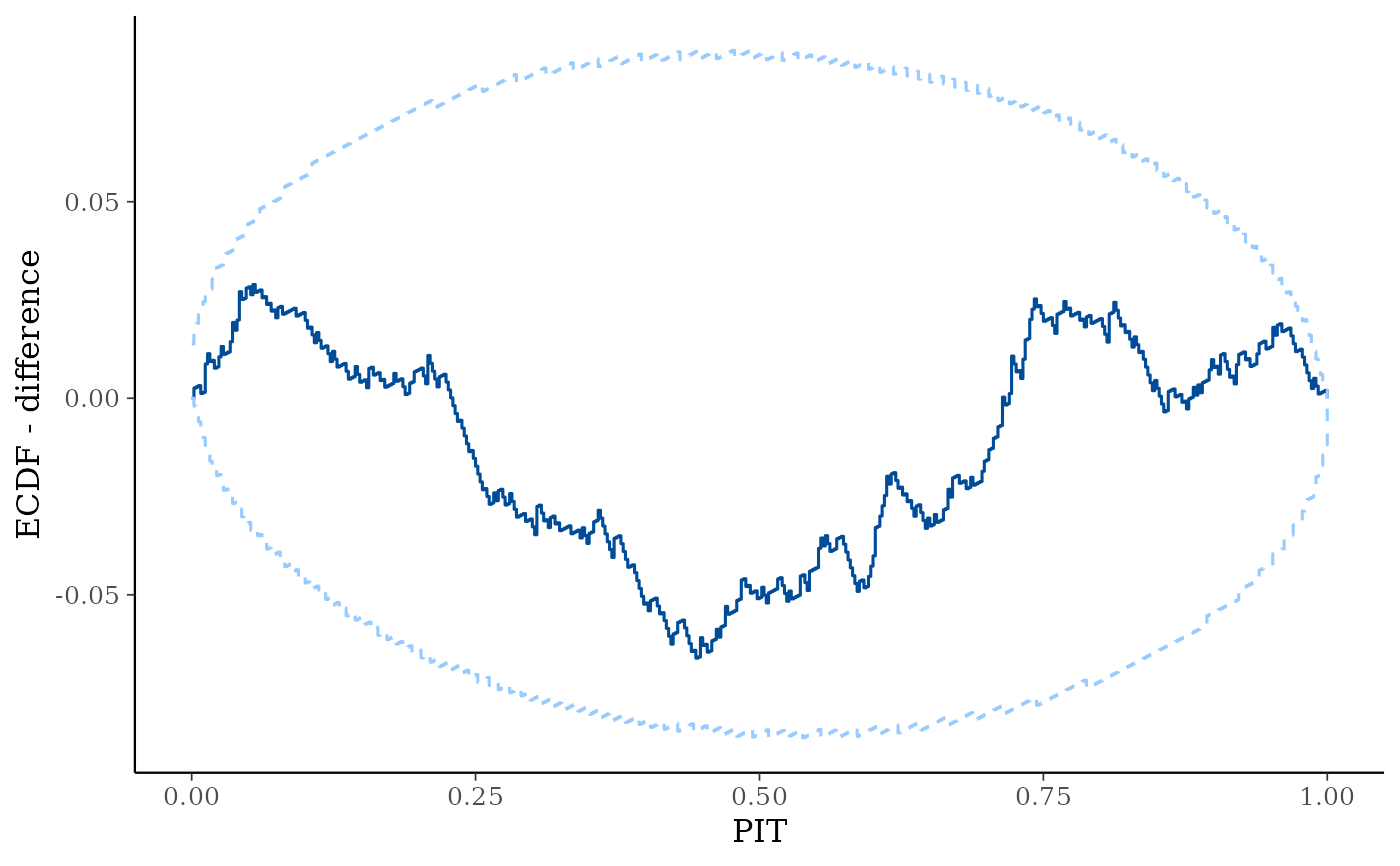# wizard hat plot
color_scheme_set("blue")
ppc_dens(y, yrep[200:202, ])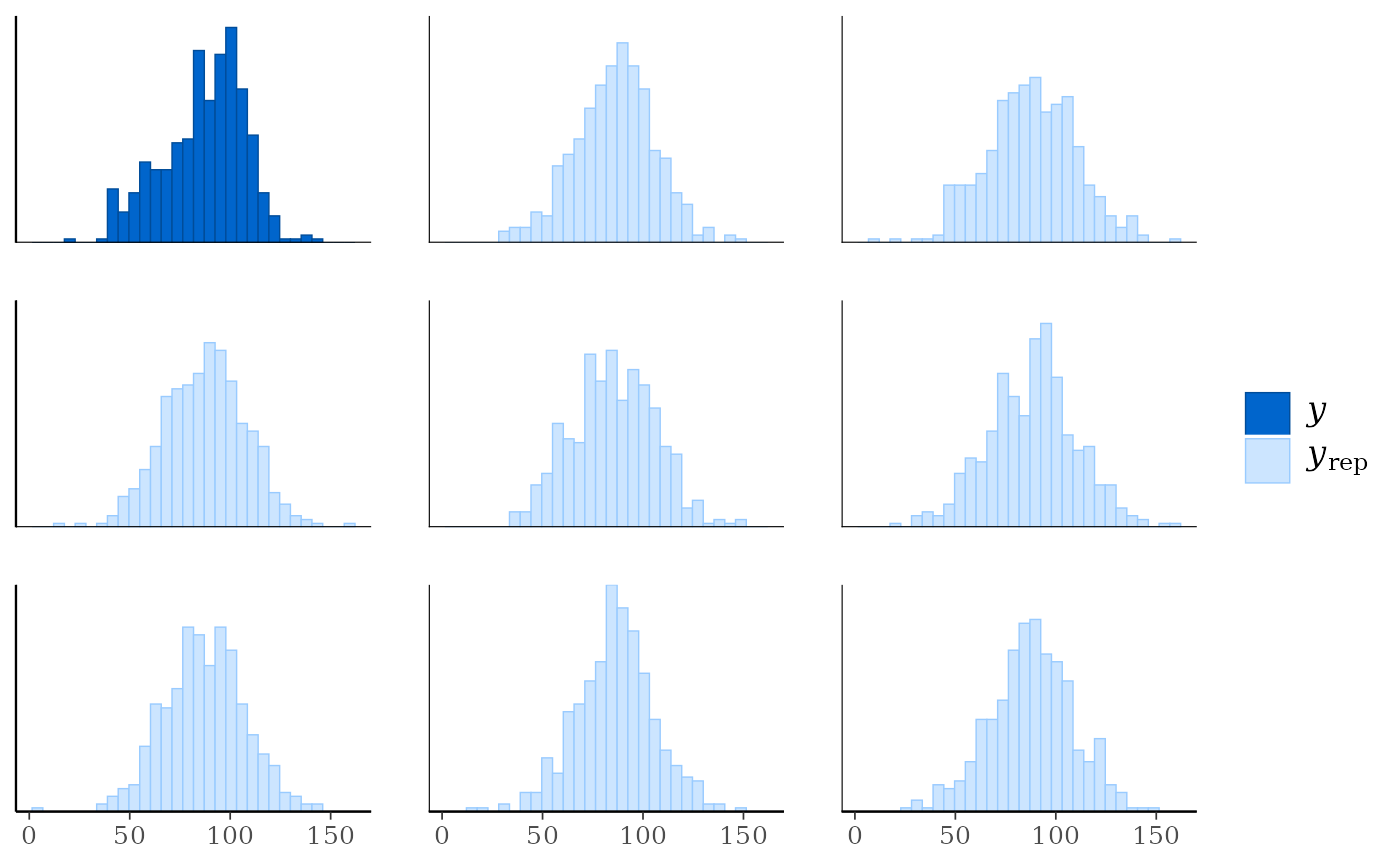ppc_freqpoly(y, yrep[1:3,], alpha = 0.1, size = 1, binwidth = 5)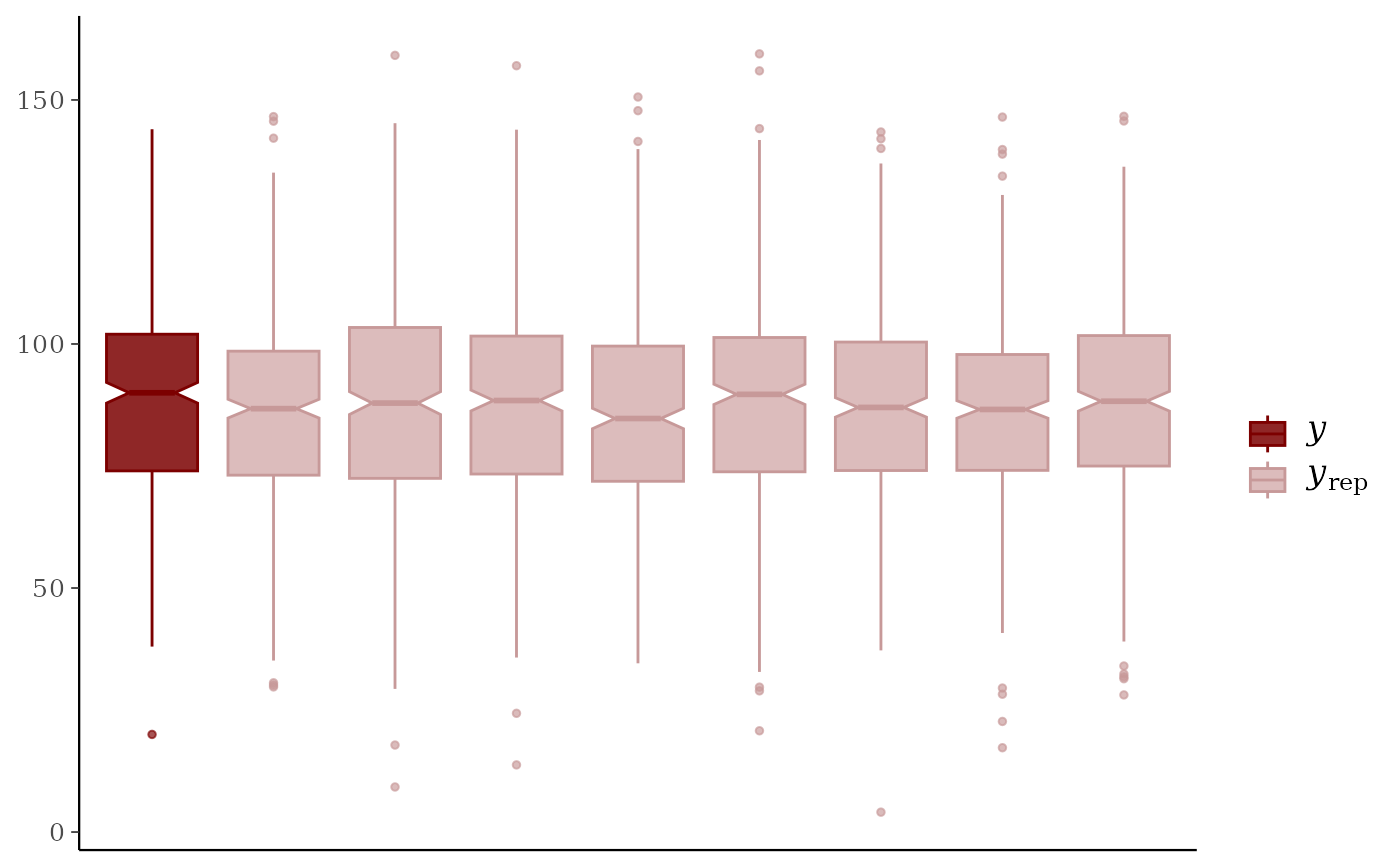# if groups are different sizes then the 'freq' argument can be useful
group <- example_group_data()
ppc_freqpoly_grouped(y, yrep[1:3,], group) + yaxis_text()#> stat_bin() using bins = 30. Pick better value with binwidth.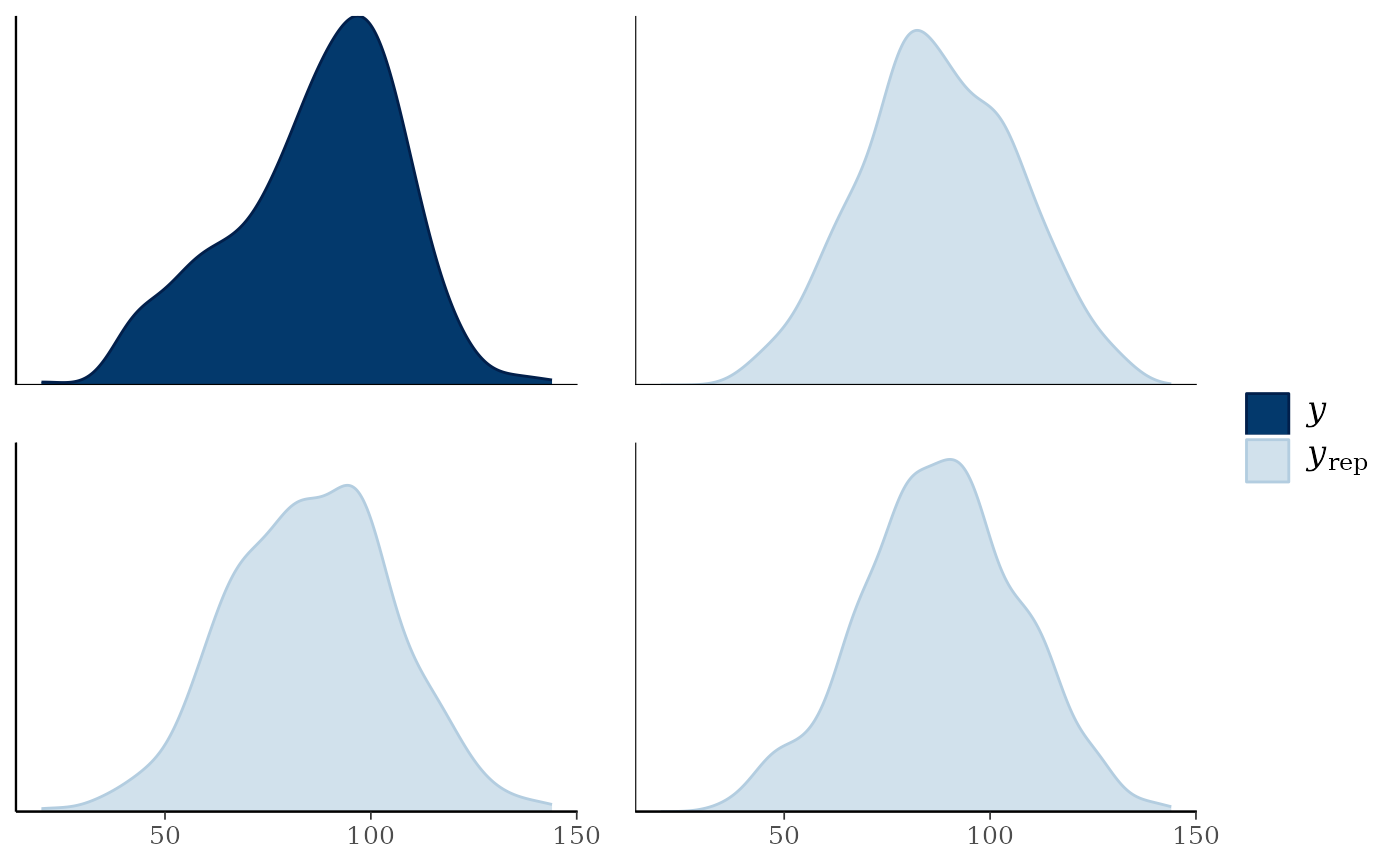ppc_freqpoly_grouped(y, yrep[1:3,], group, freq = FALSE) + yaxis_text()#> stat_bin() using bins = 30. Pick better value with binwidth.# don't need to only use small number of rows for ppc_violin_grouped
# (as it pools yrep draws within groups)
color_scheme_set("gray")
ppc_violin_grouped(y, yrep, group, size = 1.5)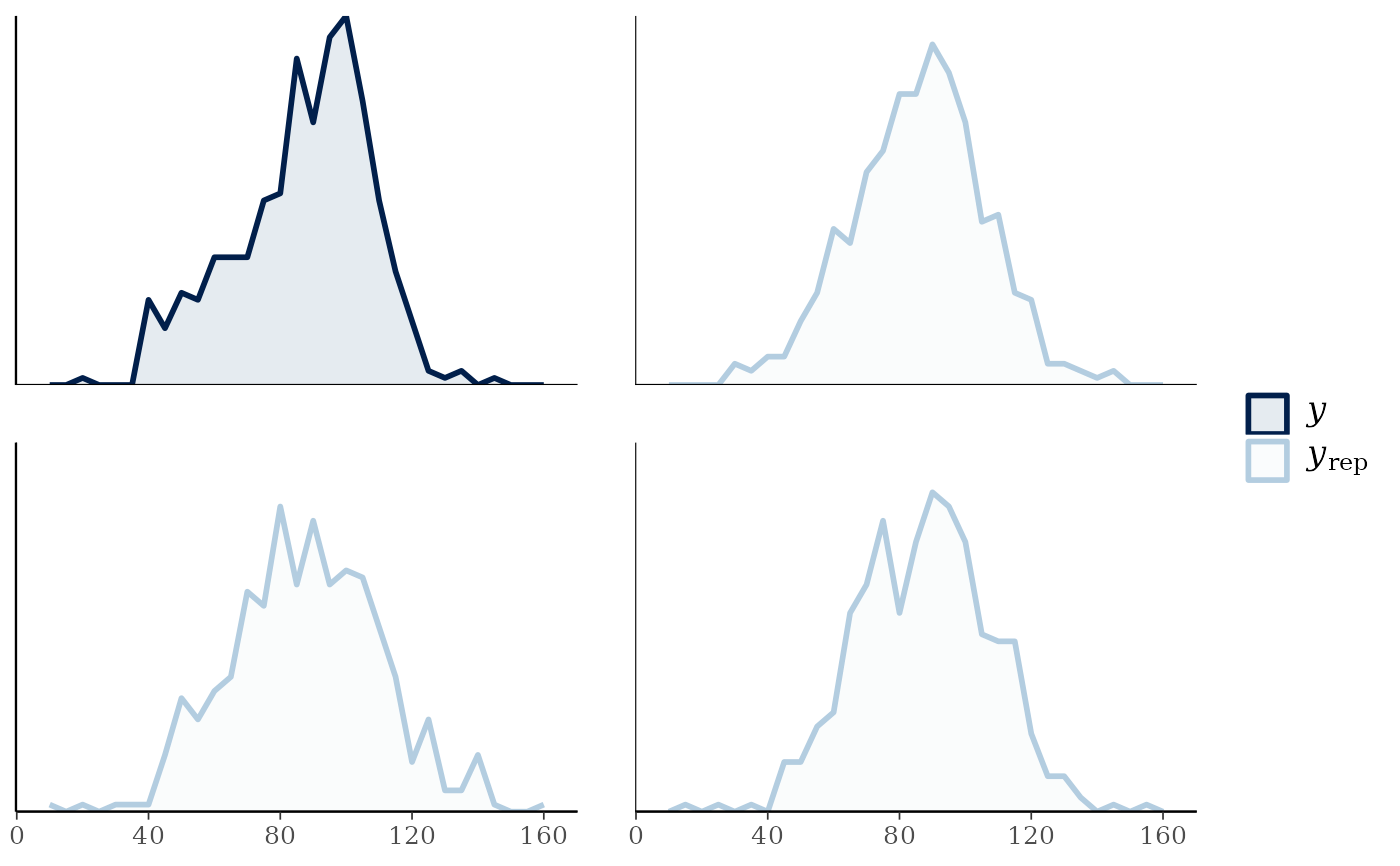ppc_violin_grouped(y, yrep, group, alpha = 0)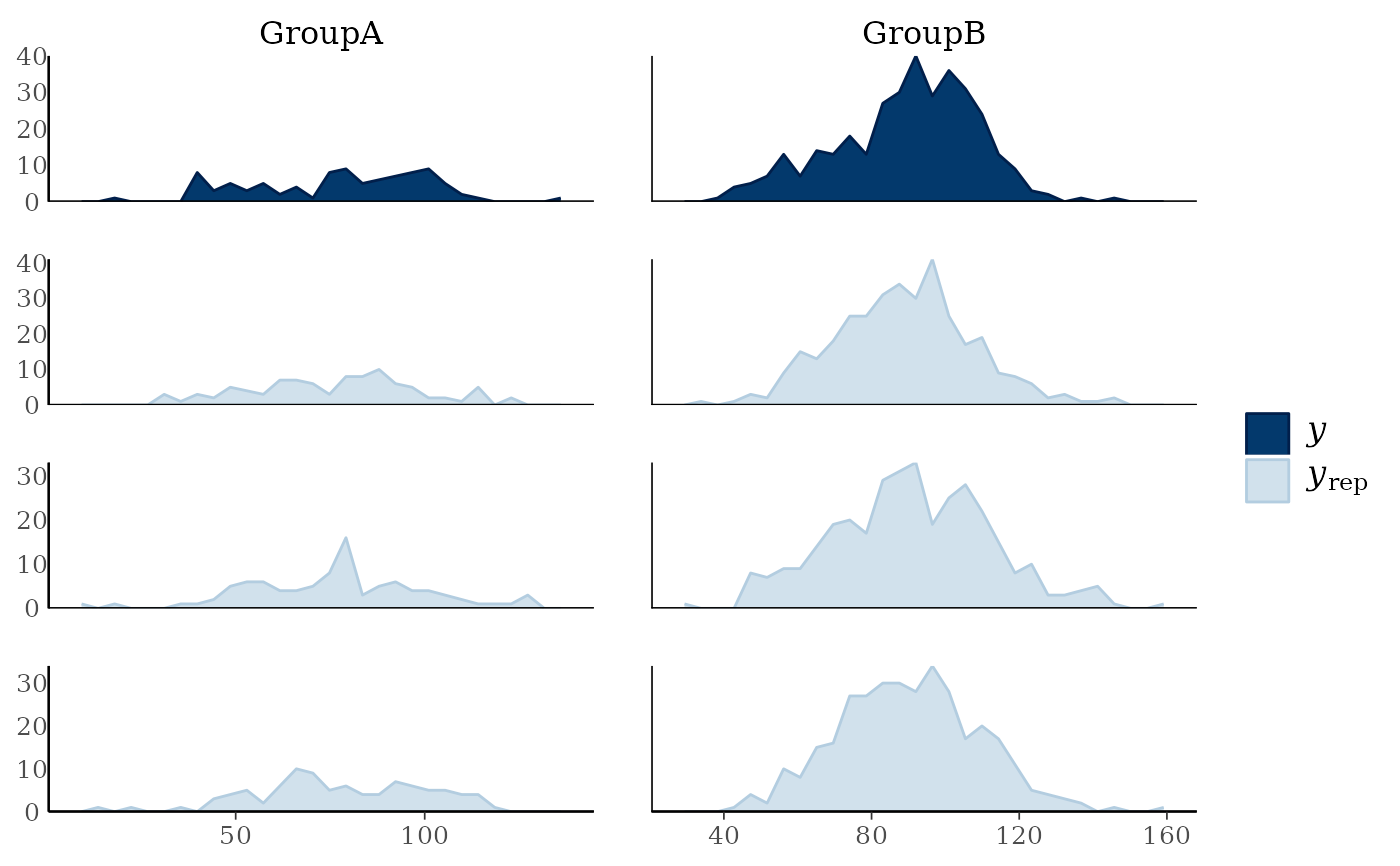# change how y is drawn
ppc_violin_grouped(y, yrep, group, alpha = 0, y_draw = "points", y_size = 1.5)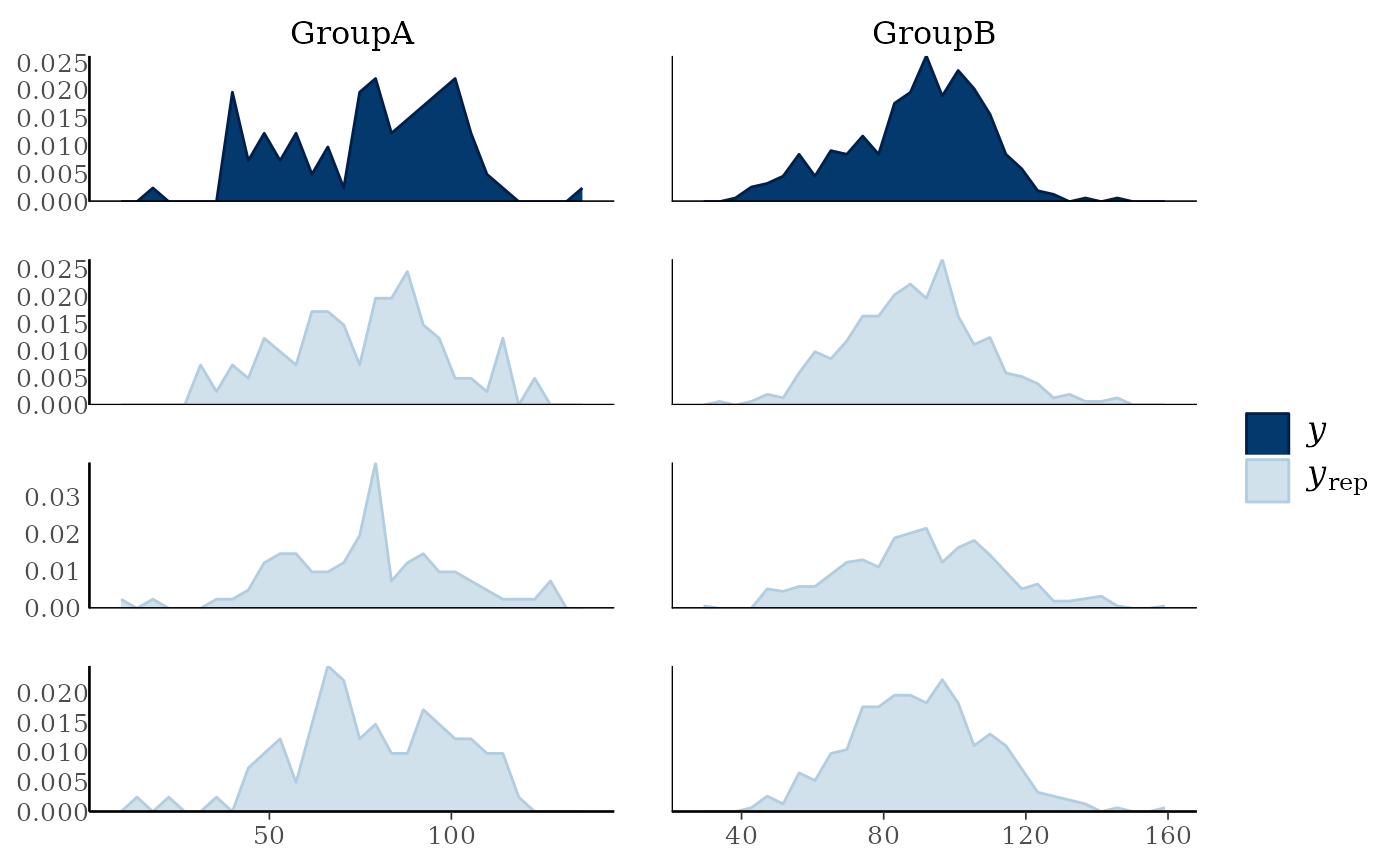ppc_violin_grouped(y, yrep, group, alpha = 0, y_draw = "both",
y_size = 1.5, y_alpha = 0.5, y_jitter = 0.33)